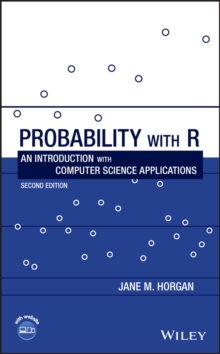Supporting your high street Find out how »
• My Account# Probability with R : An Introduction with Computer Science Applications Hardback

#### Description

Provides a comprehensive introduction to probability with an emphasis on computing-related applications This self-contained new and extended edition outlines a first course in probability applied to computer-related disciplines.

As in the first edition, experimentation and simulation are favoured over mathematical proofs.

The freely down-loadable statistical programming language R is used throughout the text, not only as a tool for calculation and data analysis, but also to illustrate concepts of probability and to simulate distributions.

The examples in Probability with R: An Introduction with Computer Science Applications, Second Edition cover a wide range of computer science applications, including: testing program performance; measuring response time and CPU time; estimating the reliability of components and systems; evaluating algorithms and queuing systems. Chapters cover: The R language; summarizing statistical data; graphical displays; the fundamentals of probability; reliability; discrete and continuous distributions; and more. This second edition includes: improved R code throughout the text, as well as new procedures, packages and interfaces;updated and additional examples, exercises and projects covering recent developments of computing;an introduction to bivariate discrete distributions together with the R functions used to handle large matrices of conditional probabilities, which are often needed in machine translation;an introduction to linear regression with particular emphasis on its application to machine learning using testing and training data;a new section on spam filtering using Bayes theorem to develop the filters;an extended range of Poisson applications such as network failures, website hits, virus attacks and accessing the cloud;use of new allocation functions in R to deal with hash table collision, server overload and the general allocation problem. The book is supplemented with a Wiley Book Companion Site featuring data and solutions to exercises within the book. Primarily addressed to students of computer science and related areas, Probability with R: An Introduction with Computer Science Applications, Second Edition is also an excellent text for students of engineering and the general sciences.

Computing professionals who need to understand the relevance of probability in their areas of practice will find it useful.

#### Information

• Format: Hardback
• Pages: 496 pages
• Publisher: John Wiley and Sons Ltd
• Publication Date:
• Category: Probability & statistics
• ISBN: 9781119536949

£89.50

£75.45